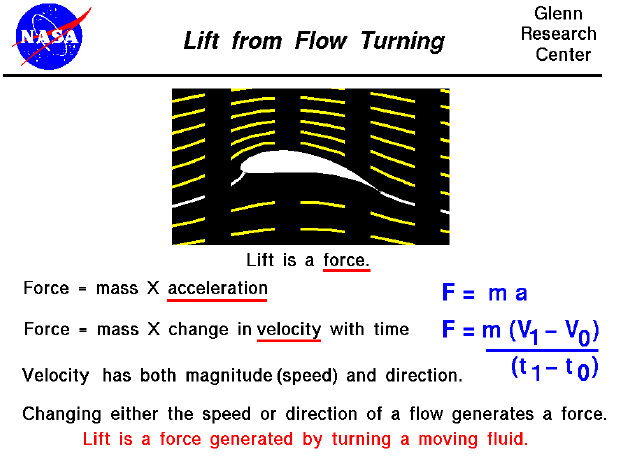Lift can be generated by a wide variety of objects, including airplane wings, rotating cylinders, spinning balls, and flat plates. Lift is the force that holds an aircraft in the air. Lift can be generated by any part of the airplane, but most of the lift on a normal airliner is generated by the wings. How is lift generated?

Force = Mass x Acceleration

Lift is a force. From Newton's Second Law of Motion, a force is produced when a mass is accelerated (F = m a). An acceleration is a change in velocity with a change in time. We have written a difference equation on this figure, but it is recognized that the relation is actually a differential (from calculus). The important fact is that a force will cause a change in velocity; and, likewise, a change in velocity will generate a force. The equation works both ways. A velocity has both a magnitude (called the speed) and a direction associated with it. Scientists and mathematicians call this a vector quantity (magnitude plus direction). So, to change either the speed or the direction of a flow, you must impose a force. And if either the speed or the direction of a flow is changed, a force will be generated.

Lift Generated in a Moving Fluid

For a body immersed in a moving fluid, the fluid will remain in contact with the surface of the body. If the body is shaped, moved, or inclined in such a way as to produce a net deflection (or turning) of the flow, the local velocity is changed in magnitude, direction, or both. Changing the velocity creates a net force on the body. It is very important to note that the turning of the fluid occurs because the molecules of the fluid stay in contact with the solid body because the molecules are free to move. Any part of the solid body can deflect a flow. Parts facing the oncoming flow are said to be windward, and parts facing away from the flow are said to be leeward. Both windward and leeward parts can deflect a flow. Ignoring the leeward deflection leads to a popular incorrect theory of lift.

Interactive Simulator

Let's investigate how lift is generated by flow turning by using a Java simulator.

Here we see a yellow flat plate immersed in a flow of air. The air appears as small blue and white particle traces which move from left to right. The plate is inclined at an angle and notice that both the flow above and below the plate are turned along the plate. The white lines are the streamlines which intersect the plate and are called stagnation streamlines. You can vary the angle of the plate by using the slider below the view window or by backspacing over the input box, typing in your new value and hitting the Enter key on the keyboard. On the right side of the simulator is a gage with some buttons and some sliders. The gage tells you the value of the velocity or pressure at the location of the probe (little purple dot) in the left view window. You can change the location from side to side by using the slider located below the gage, and you can change the location up and down by using the slider to the left of the gage. You select which variable to display by using the white buttons labeled Velocity, Pressure, or Smoke. Smoke causes green particles to be released from the probe. The blue buttons control the type of display shown in the left view window.

You can further investigate the effect of airfoil shape and the other factors affecting lift by using the FoilSim II Java Applet. You can also download your own copy of FoilSim to play with for free.

Changes in Speed or Direction

Lift is a force generated by turning a flow. Since a force is a vector quantity (like the velocity), it has both a magnitude and a direction. The direction of the lift force is defined to be perpendicular to the initial flow direction. (The drag is defined to be along the flow direction.) The magnitude depends on several factors concerning the object and the flow.

Summary

Lift and drag are mechanical forces generated on the surface of an object as it interacts with a fluid. The net fluid force is generated by the pressure acting over the entire surface of a closed body. The pressure varies around a body in a moving fluid because it is related to the fluid momentum (mass times velocity). The velocity varies around the body because of the flow deflection described above.Guided Tours